Anzeige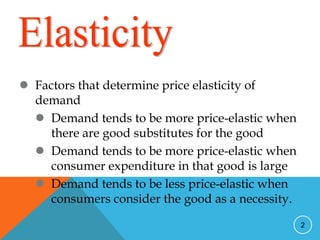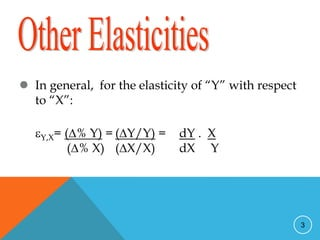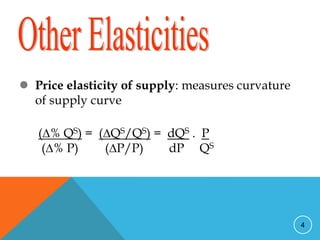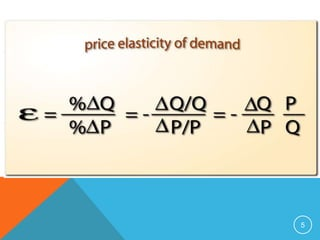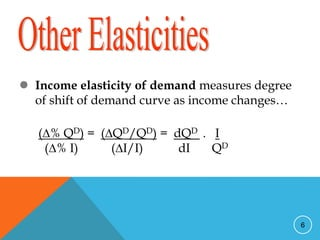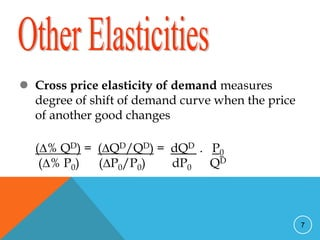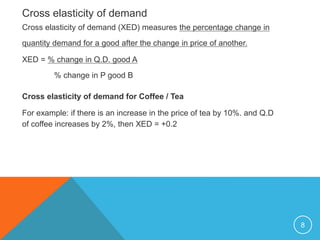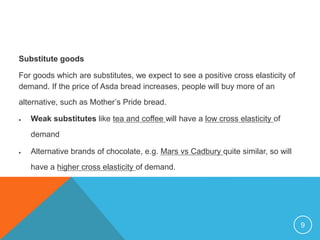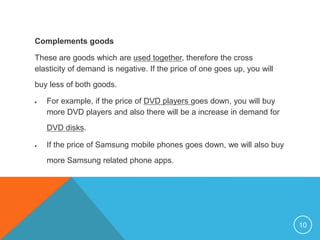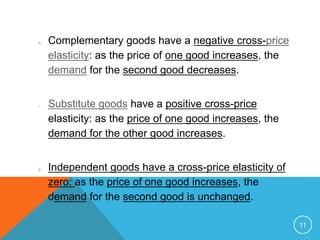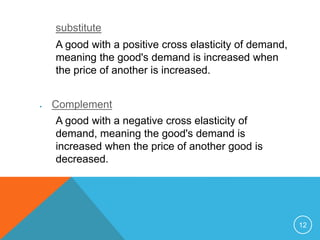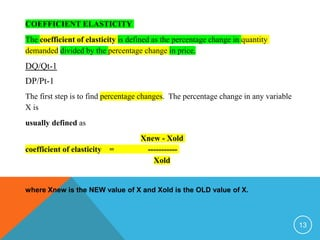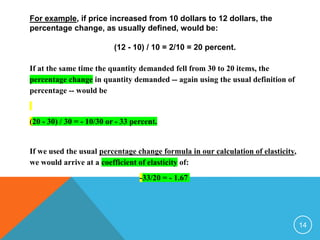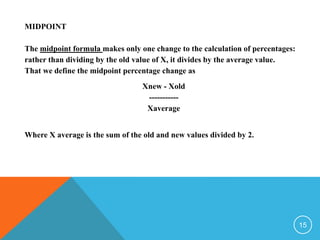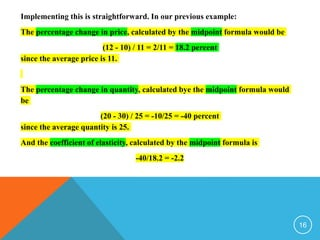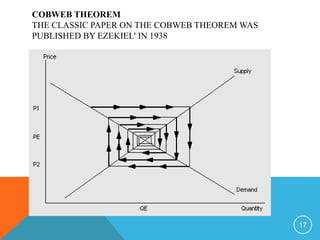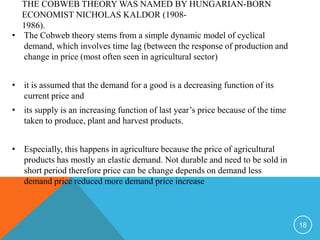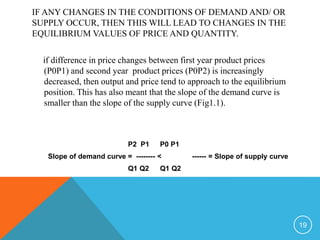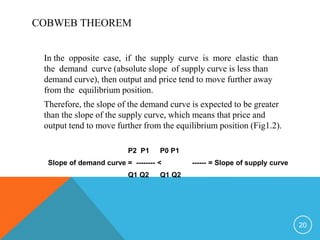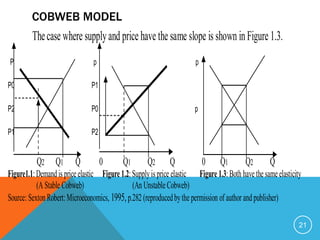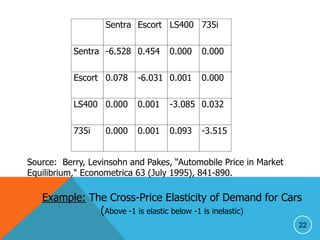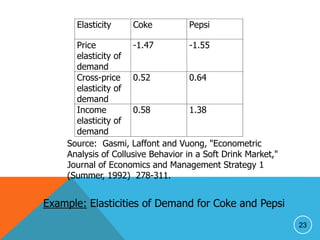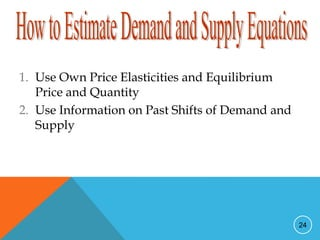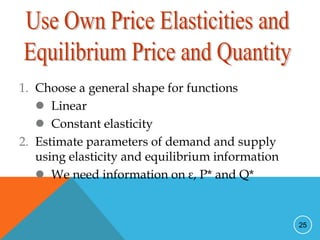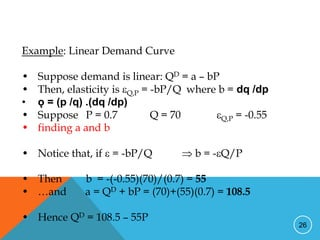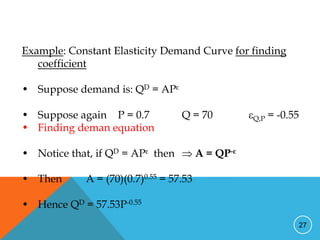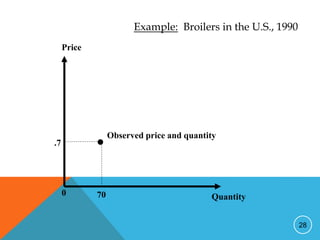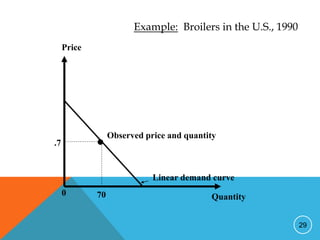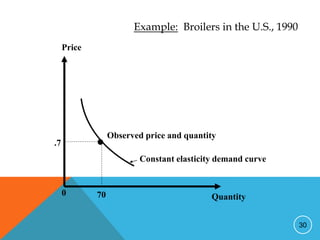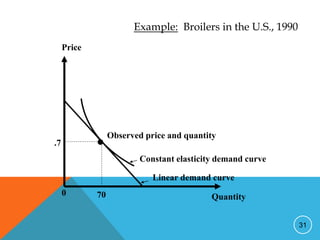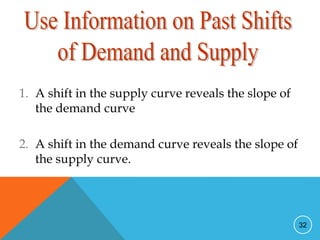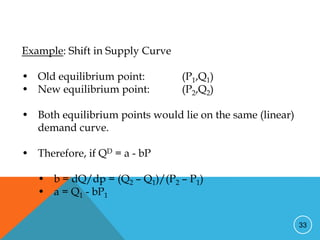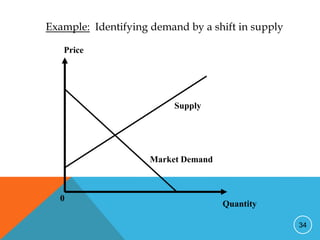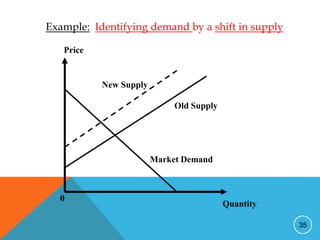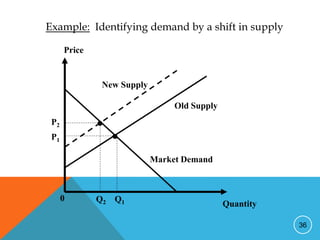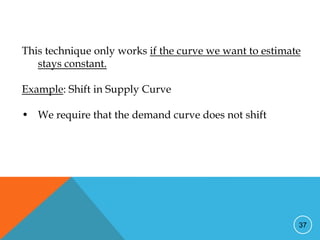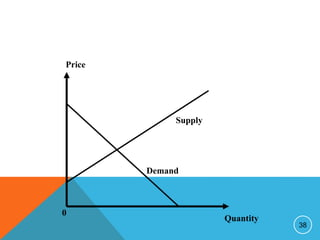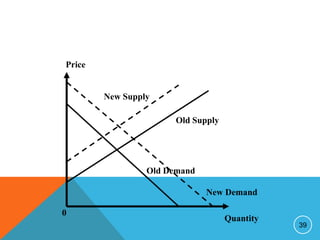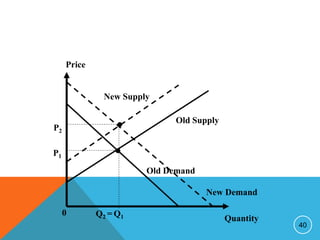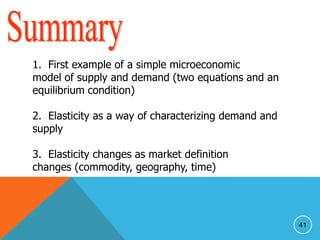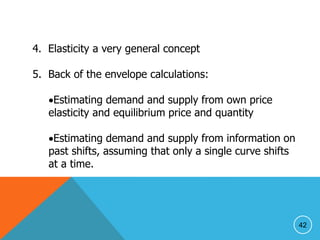1 von 42
Anzeige

### Ch 2 supply demand and elasticity-2.2.ppt

1. Demand and Supply cont 2 Lecturer: Assoc Prof Akalpler
2. 2  Factors that determine price elasticity of demand  Demand tends to be more price-elastic when there are good substitutes for the good  Demand tends to be more price-elastic when consumer expenditure in that good is large  Demand tends to be less price-elastic when consumers consider the good as a necessity.
3. 3  In general, for the elasticity of “Y” with respect to “X”: Y,X= (% Y) = (Y/Y) = dY . X (% X) (X/X) dX Y
4. 4  Price elasticity of supply: measures curvature of supply curve (% QS) = (QS/QS) = dQS . P (% P) (P/P) dP QS
5. 5
6. 6  Income elasticity of demand measures degree of shift of demand curve as income changes… (% QD) = (QD/QD) = dQD . I (% I) (I/I) dI QD
7. 7  Cross price elasticity of demand measures degree of shift of demand curve when the price of another good changes (% QD) = (QD/QD) = dQD . P0 (% P0) (P0/P0) dP0 QD
8. 8 Cross elasticity of demand Cross elasticity of demand (XED) measures the percentage change in quantity demand for a good after the change in price of another. XED = % change in Q.D. good A % change in P good B Cross elasticity of demand for Coffee / Tea For example: if there is an increase in the price of tea by 10%. and Q.D of coffee increases by 2%, then XED = +0.2
9. 9 Substitute goods For goods which are substitutes, we expect to see a positive cross elasticity of demand. If the price of Asda bread increases, people will buy more of an alternative, such as Mother’s Pride bread.  Weak substitutes like tea and coffee will have a low cross elasticity of demand  Alternative brands of chocolate, e.g. Mars vs Cadbury quite similar, so will have a higher cross elasticity of demand.
10. 10 Complements goods These are goods which are used together, therefore the cross elasticity of demand is negative. If the price of one goes up, you will buy less of both goods.  For example, if the price of DVD players goes down, you will buy more DVD players and also there will be a increase in demand for DVD disks.  If the price of Samsung mobile phones goes down, we will also buy more Samsung related phone apps.
11. 11 o Complementary goods have a negative cross-price elasticity: as the price of one good increases, the demand for the second good decreases. o Substitute goods have a positive cross-price elasticity: as the price of one good increases, the demand for the other good increases. o Independent goods have a cross-price elasticity of zero: as the price of one good increases, the demand for the second good is unchanged.
12. 12 substitute A good with a positive cross elasticity of demand, meaning the good's demand is increased when the price of another is increased.  Complement A good with a negative cross elasticity of demand, meaning the good's demand is increased when the price of another good is decreased.
13. 13 COEFFICIENT ELASTICITY The coefficient of elasticity is defined as the percentage change in quantity demanded divided by the percentage change in price. DQ/Qt-1 DP/Pt-1 The first step is to find percentage changes. The percentage change in any variable X is usually defined as Xnew - Xold coefficient of elasticity = ----------- Xold where Xnew is the NEW value of X and Xold is the OLD value of X.
14. 14 For example, if price increased from 10 dollars to 12 dollars, the percentage change, as usually defined, would be: (12 - 10) / 10 = 2/10 = 20 percent. If at the same time the quantity demanded fell from 30 to 20 items, the percentage change in quantity demanded -- again using the usual definition of percentage -- would be (20 - 30) / 30 = - 10/30 or - 33 percent. If we used the usual percentage change formula in our calculation of elasticity, we would arrive at a coefficient of elasticity of: -33/20 = - 1.67
15. 15 MIDPOINT The midpoint formula makes only one change to the calculation of percentages: rather than dividing by the old value of X, it divides by the average value. That we define the midpoint percentage change as Xnew - Xold ----------- Xaverage Where X average is the sum of the old and new values divided by 2.
16. 16 Implementing this is straightforward. In our previous example: The percentage change in price, calculated by the midpoint formula would be (12 - 10) / 11 = 2/11 = 18.2 percent since the average price is 11. The percentage change in quantity, calculated bye the midpoint formula would be (20 - 30) / 25 = -10/25 = -40 percent since the average quantity is 25. And the coefficient of elasticity, calculated by the midpoint formula is -40/18.2 = -2.2
17. COBWEB THEOREM THE CLASSIC PAPER ON THE COBWEB THEOREM WAS PUBLISHED BY EZEKIEL' IN 1938 17
18. THE COBWEB THEORY WAS NAMED BY HUNGARIAN-BORN ECONOMIST NICHOLAS KALDOR (1908- 1986). • The Cobweb theory stems from a simple dynamic model of cyclical demand, which involves time lag (between the response of production and change in price (most often seen in agricultural sector) • it is assumed that the demand for a good is a decreasing function of its current price and • its supply is an increasing function of last year’s price because of the time taken to produce, plant and harvest products. • Especially, this happens in agriculture because the price of agricultural products has mostly an elastic demand. Not durable and need to be sold in short period therefore price can be change depends on demand less demand price reduced more demand price increase 18
19. IF ANY CHANGES IN THE CONDITIONS OF DEMAND AND/ OR SUPPLY OCCUR, THEN THIS WILL LEAD TO CHANGES IN THE EQUILIBRIUM VALUES OF PRICE AND QUANTITY. if difference in price changes between first year product prices (P0P1) and second year product prices (P0P2) is increasingly decreased, then output and price tend to approach to the equilibrium position. This has also meant that the slope of the demand curve is smaller than the slope of the supply curve (Fig1.1). P2 P1 P0 P1 Slope of demand curve = -------- < ------ = Slope of supply curve Q1 Q2 Q1 Q2 19
20. COBWEB THEOREM In the opposite case, if the supply curve is more elastic than the demand curve (absolute slope of supply curve is less than demand curve), then output and price tend to move further away from the equilibrium position. Therefore, the slope of the demand curve is expected to be greater than the slope of the supply curve, which means that price and output tend to move further from the equilibrium position (Fig1.2). P2 P1 P0 P1 Slope of demand curve = -------- < ------ = Slope of supply curve Q1 Q2 Q1 Q2 20
21. COBWEB MODEL Thecase where supplyand price have the same slope is shown inFigure 1.3. P p p P0 P1 P2 P0 p P1 P2 Q2 Q1 Q 0 Q1 Q2 Q 0 Q1 Q2 Q Figure1.1:Demandispriceelastic Figure1.2:Supplyisprice elastic Figure1.3:Both havethesameelasticity (A Stable Cobweb) (An UnstableCobweb) Source: SextonRobert:Microeconomics, 1995,p.282 (reproducedbythe permission of author andpublisher) 21
22. 22 Sentra Escort LS400 735i Sentra -6.528 0.454 0.000 0.000 Escort 0.078 -6.031 0.001 0.000 LS400 0.000 0.001 -3.085 0.032 735i 0.000 0.001 0.093 -3.515 Source: Berry, Levinsohn and Pakes, “Automobile Price in Market Equilibrium," Econometrica 63 (July 1995), 841-890. Example: The Cross-Price Elasticity of Demand for Cars (Above -1 is elastic below -1 is inelastic)
23. 23 Elasticity Coke Pepsi Price elasticity of demand -1.47 -1.55 Cross-price elasticity of demand 0.52 0.64 Income elasticity of demand 0.58 1.38 Source: Gasmi, Laffont and Vuong, "Econometric Analysis of Collusive Behavior in a Soft Drink Market," Journal of Economics and Management Strategy 1 (Summer, 1992) 278-311. Example: Elasticities of Demand for Coke and Pepsi
24. 24 1. Use Own Price Elasticities and Equilibrium Price and Quantity 2. Use Information on Past Shifts of Demand and Supply
25. 25 1. Choose a general shape for functions  Linear  Constant elasticity 2. Estimate parameters of demand and supply using elasticity and equilibrium information  We need information on ε, P* and Q*
26. 26 Example: Linear Demand Curve • Suppose demand is linear: QD = a – bP • Then, elasticity is Q,P = -bP/Q where b = dq /dp • ǫ = (p /q) .(dq /dp) • Suppose P = 0.7 Q = 70 Q,P = -0.55 • finding a and b • Notice that, if  = -bP/Q  b = -Q/P • Then b = -(-0.55)(70)/(0.7) = 55 • …and a = QD + bP = (70)+(55)(0.7) = 108.5 • Hence QD = 108.5 – 55P
27. 27 Example: Constant Elasticity Demand Curve for finding coefficient • Suppose demand is: QD = APε • Suppose again P = 0.7 Q = 70 Q,P = -0.55 • Finding deman equation • Notice that, if QD = APε then  A = QP-ε • Then A = (70)(0.7)0.55 = 57.53 • Hence QD = 57.53P-0.55
28. 28 Quantity Price 0 70 .7 • Observed price and quantity Example: Broilers in the U.S., 1990
29. 29 Quantity Price 0 70 .7 • Observed price and quantity Linear demand curve Example: Broilers in the U.S., 1990
30. 30 Quantity Price 0 70 .7 • Observed price and quantity Constant elasticity demand curve Example: Broilers in the U.S., 1990
31. 31 Quantity Price 0 70 .7 • Observed price and quantity Constant elasticity demand curve Linear demand curve Example: Broilers in the U.S., 1990
32. 32 1. A shift in the supply curve reveals the slope of the demand curve 2. A shift in the demand curve reveals the slope of the supply curve.
33. 33 Example: Shift in Supply Curve • Old equilibrium point: (P1,Q1) • New equilibrium point: (P2,Q2) • Both equilibrium points would lie on the same (linear) demand curve. • Therefore, if QD = a - bP • b = dQ/dp = (Q2 – Q1)/(P2 – P1) • a = Q1 - bP1
34. 34 Quantity Price 0 Market Demand Supply Example: Identifying demand by a shift in supply
35. 35 Quantity Price 0 Market Demand New Supply Old Supply Example: Identifying demand by a shift in supply
36. 36 Quantity Price 0 Market Demand New Supply Q2 • • Q1 Old Supply P2 P1 Example: Identifying demand by a shift in supply
37. 37 This technique only works if the curve we want to estimate stays constant. Example: Shift in Supply Curve • We require that the demand curve does not shift
38. 38 Quantity Price 0 Demand Supply
39. 39 Quantity Price 0 Old Demand New Supply Old Supply New Demand
40. 40 Quantity Price 0 Old Demand New Supply Q2 = • • Q1 Old Supply P2 P1 New Demand
41. 41 1. First example of a simple microeconomic model of supply and demand (two equations and an equilibrium condition) 2. Elasticity as a way of characterizing demand and supply 3. Elasticity changes as market definition changes (commodity, geography, time)
42. 42 4. Elasticity a very general concept 5. Back of the envelope calculations: Estimating demand and supply from own price elasticity and equilibrium price and quantity Estimating demand and supply from information on past shifts, assuming that only a single curve shifts at a time.
Anzeige